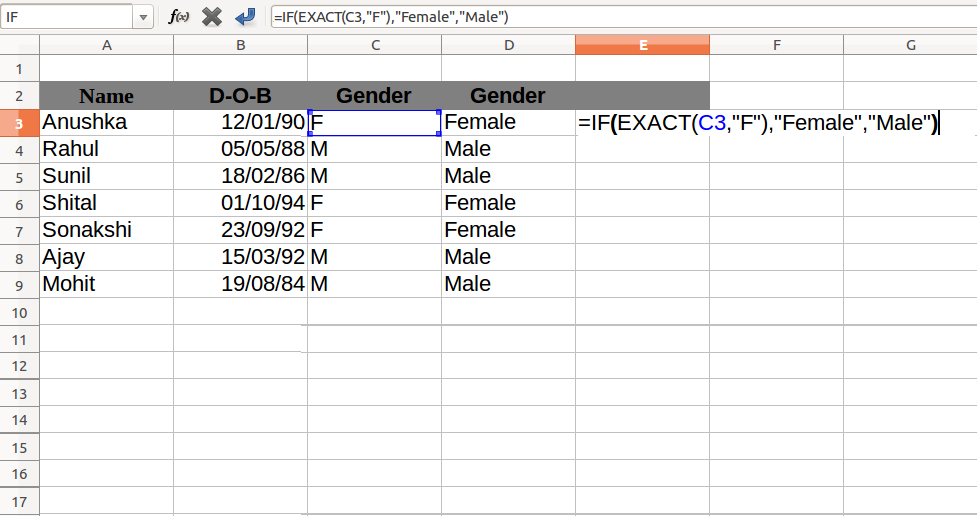Exact function in Excel : In this tutorial, we will learn about syntax and usage of the Exact function.

## Description :

Exact function in excel is used to compare two strings (text) and returns TRUE if the string is same, otherwise return FALSE. Exact function is case sensitive so small and capital letters are not equal.

## Syntax:

EXACT(text1,text2)

• text1 – first string or excel cell reference that contains a text.
• text2 – second string or excel cell reference that contains a text.

## Note :

Based on the above example EXACT function returns FALSE because ‘a’ and ‘A’ are different (EXACT function is case-sensitive).

## Exact With IF Function

We can also use the exact function with IF as a condition because the Exact function returns TRUE or FALSE. You can learn detail about IF Function Here. Following example explain it in detail.Above example show gender of a person, based on given abbreviation. As we know IF accepts TRUE or FALSE as a condition then perform a task based on given result and, the EXACT function returns TRUE if Text is Equal otherwise returns FALSE.

EXACT Function in Excel